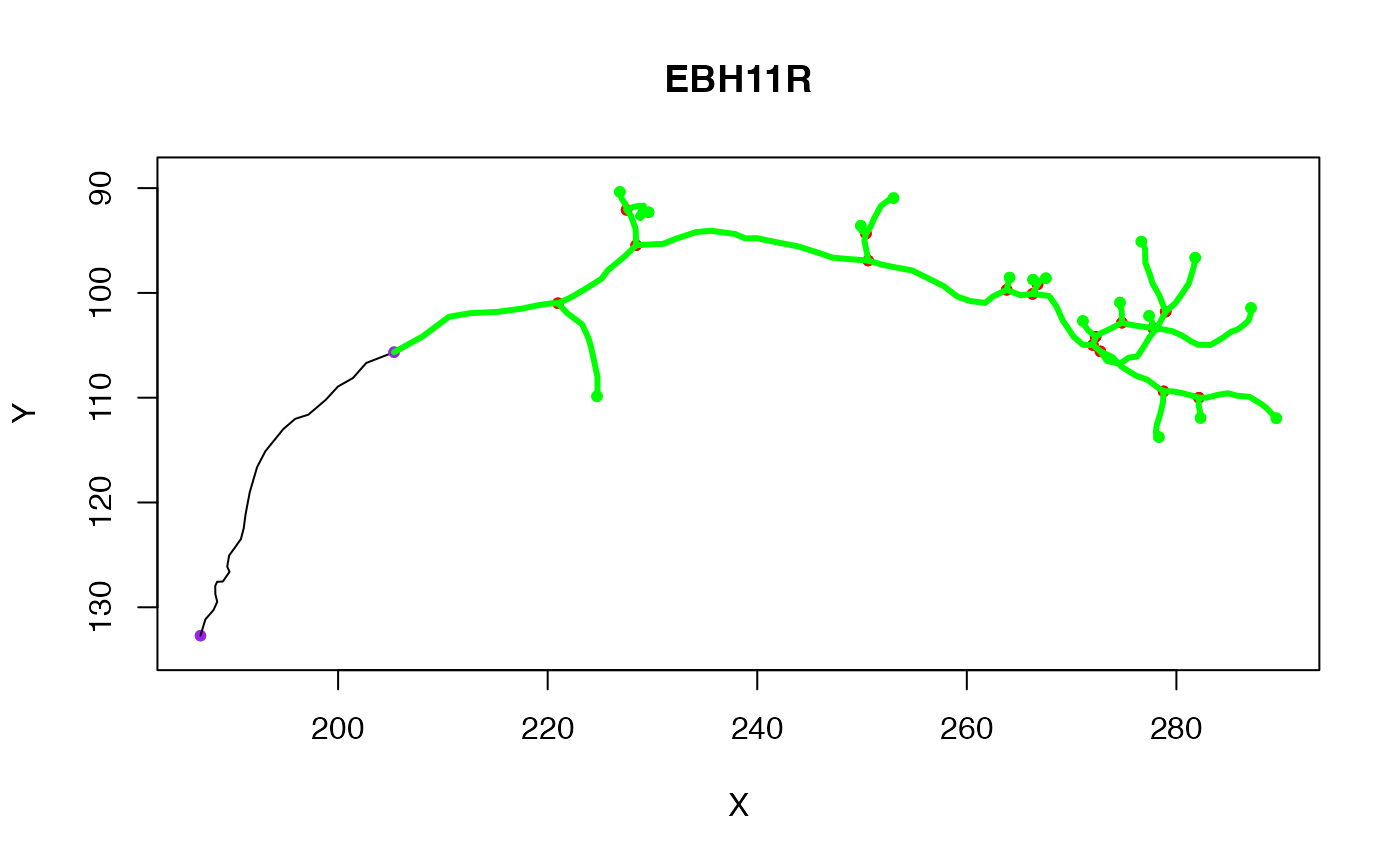prune_vertices removes vertices from a neuron

prune_edges removes edges (and any unreferenced vertices)

prune_vertices(x, verticestoprune, invert = FALSE, ...)

prune_edges(x, edges, invert = FALSE, ...)

## Arguments

x

A neuron to prune. This can be any object that can be converted by as.ngraph --- see details.

verticestoprune

An integer vector describing which vertices to remove.

invert

Whether to keep vertices rather than dropping them (default FALSE).

...

Additional arguments passed to as.neuron.ngraph

edges

The edges to remove. One of i) an Nx2 matrix, each row specifying a single edge defined by its raw edge id, ii) an integer vector defining a path of raw vertex ids or iii) an igraph.es edge sequence --- see details and the P and path arguments of igraph::E.

## Value

A pruned neuron

## Details

These are relatively low-level functions and you will probably want to use subset.neuron or prune.neuron and friends in many cases.

Note that prune_vertices and prune_edges both use raw vertex ids to specify the vertices/edges to be removed. If you want to use the id in the PointNo field, then you must translate yourself (see examples).

Both prune_vertices and prune_edges first convert their input x to the ngraph representation of the neuron before removing points. The input x can therefore be in any form compatible with as.ngraph including an existing ngraph. There is an additional requirement that the input must be compatible with xyzmatrix if invert=TRUE.

Note that the edges argument of prune_edges must specify a path traversing a set of vertices in a valid order. However if the input is a matrix or vector the direction of each individual edge in this path is ignored. So if your neuron has edges 2->1 2->3 3->4 then an edge sequence 1:3 would successfully delete 2 edges.

as.neuron.ngraph, subset.neuron, prune.neuron

## Examples

n=prune_vertices(Cell07PNs[], 1:25)
# original neuron
plot(Cell07PNs[])
# with pruned neuron superimposed# use the PointNo field (= the original id from an SWC file)
n2=prune_vertices(n, match(26:30, n$d$PointNo))
y=prune_edges(Cell07PNs[], edges=1:25)

# remove the spine of a neuron
spine_ids=spine(Cell07PNs[], rval='ids')
pruned=prune_edges(Cell07PNs[], spine_ids)

# NB this is subtly different from this, which removes vertices along the
# spine *even* if they are part of an edge that is outside the spine.
pruned2=prune_vertices(Cell07PNs[], spine_ids)